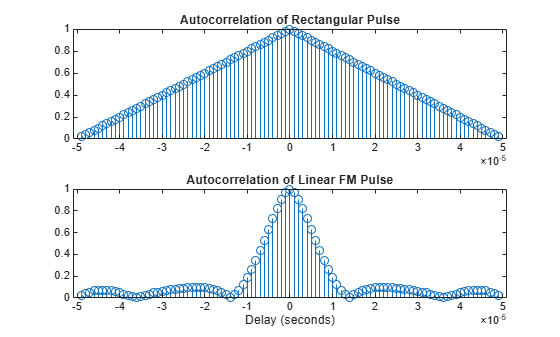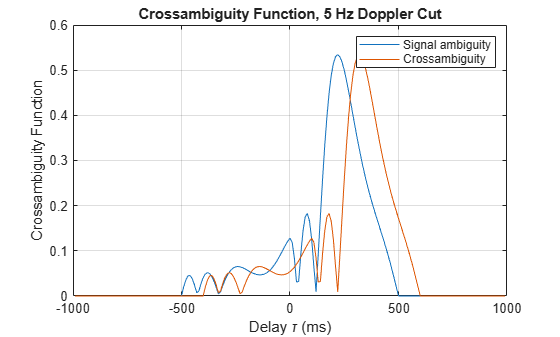# ambgfun

Ambiguity and crossambiguity function

## Syntax

afmag = ambgfun(x,Fs,PRF) afmag = ambgfun(x,y,Fs,PRF) [afmag,delay,doppler] = ambgfun(___) [afmag,delay,doppler] = ambgfun(___,'Cut','2D') [afmag,delay] = ambgfun(___,'Cut','Doppler') [afmag,delay] = ambgfun(___,'Cut','Doppler','CutValue',V) [afmag,doppler] = ambgfun(___,'Cut','Delay') [afmag,doppler] = ambgfun(___,'Cut','Delay','CutValue',V) ambgfun(___) 

## Description

afmag = ambgfun(x,Fs,PRF) returns the magnitude of the normalized ambiguity function for the vector x. Fs is the sampling rate. PRF is the pulse repetition rate.

afmag = ambgfun(x,y,Fs,PRF) returns the magnitude of the normalized crossambiguity function between the pulse x and the pulse y.

[afmag,delay,doppler] = ambgfun(___) or [afmag,delay,doppler] = ambgfun(___,'Cut','2D') returns the time delay vector, delay, and the Doppler frequency vector, doppler.

[afmag,delay] = ambgfun(___,'Cut','Doppler') returns delays from a zero-Doppler cut through the 2-D normalized ambiguity function magnitude.

[afmag,delay] = ambgfun(___,'Cut','Doppler','CutValue',V) returns delays from a nonzero Doppler cut through the 2-D normalized ambiguity function magnitude at Doppler value, V.

[afmag,doppler] = ambgfun(___,'Cut','Delay') returns the Doppler values from zero-delay cut through the 2-D normalized ambiguity function magnitude.

[afmag,doppler] = ambgfun(___,'Cut','Delay','CutValue',V) returns the Doppler values from a one-dimensional cut through the 2-D normalized ambiguity function magnitude at a delay value of V.

ambgfun(___), with no output arguments, plots the ambiguity or crossambiguity function. When 'Cut' is '2D', the function produces a contour plot of the periodic ambiguity function. When 'Cut' is 'Delay' or 'Doppler', the function produces a line plot of the periodic ambiguity function cut.

## Input Arguments

collapse all

Input pulse waveform.

Second input pulse waveform.

Sampling rate in hertz.

Pulse repetition frequency in hertz.

### Name-Value Pair Arguments

Specify optional comma-separated pairs of Name,Value arguments. Name is the argument name and Value is the corresponding value. Name must appear inside quotes. You can specify several name and value pair arguments in any order as Name1,Value1,...,NameN,ValueN.

Example: 'Cut','Doppler','CutValue',10 specifies that a vector of ambiguity function values be produced at a Doppler shift of 10 Hz.

Used to generate an ambiguity surface or one-dimensional cut through the ambiguity diagram. The value '2D' generates a surface plot of the two-dimensional ambiguity function. The direction of the one-dimensional cut is determined by setting the value of 'Cut' to 'Delay' or 'Doppler'.

The choice of 'Delay' generates a cut at zero time delay. In this case, the second output argument of ambgfuncontains the ambiguity function values at Doppler shifted values. You can create a cut at nonzero time delay using the name-value pair 'CutValue'.

The choice of 'Doppler' generates a cut at zero Doppler shift. In this case, the second output argument of ambgfun contains the ambiguity function values at time-delayed values. You can create cut at nonzero Doppler using the name-value pair 'CutValue'.

When setting the name-value pair 'Cut' to 'Delay' or 'Doppler', you can set 'CutValue' to specify a cross-section that may not coincide with either zero time delay or zero Doppler shift. However, 'CutValue' cannot be used when 'Cut' is set to '2D'.

When 'Cut' is set to 'Delay' ,'CutValue' is the time delay at which the cut is taken. Time delay units are in seconds.

When 'Cut' is set to 'Doppler', 'CutValue' is the Doppler shift at which the cut is taken. Doppler units are in hertz.

Example: 'CutValue',10.0

Data Types: double

## Output Arguments

 afmag Normalized ambiguity or crossambiguity function magnitudes. afmag is an M-by-N matrix where M is the number of Doppler frequencies and N is the number of time delays. delay Time delay vector. delay is an N-by-1 vector of time delays. For the ambiguity function, if Nx is the length of signal x, then the delay vector consist of N = 2Nx – 1 samples in the range, –(Nx/2) – 1,...,(Nx/2) – 1). For the crossambiguity function, let Ny be the length of the second signal. The time delay vector consists of N = Nx+ Ny– 1 equally spaced samples. For an even number of delays, the delay sample times are –(N/2 – 1)/Fs,...,(N/2 – 1))/Fs. For an odd number of delays, if Nf = floor(N/2), the delay sample times are –Nf /Fs,...,(Nf – 1)/Fs. doppler Doppler frequency vector. doppler is an M-by-1 vector of Doppler frequencies. The Doppler frequency vector consists of M = 2ceil(log2 N) equally-spaced samples. Frequencies are (–(M/2)Fs,...,(M/2–1)Fs).

## Examples

collapse all

Plot the ambiguity function magnitude of a rectangular pulse.

waveform = phased.RectangularWaveform; x = waveform(); PRF = 2e4; [afmag,delay,doppler] = ambgfun(x,waveform.SampleRate,PRF); contour(delay,doppler,afmag) xlabel('Delay (seconds)') ylabel('Doppler Shift (hertz)')This example shows how to plot zero-Doppler cuts of the autocorrelation sequences of rectangular and linear FM pulses of equal duration. Note the pulse compression exhibited in the autocorrelation sequence of the linear FM pulse.

hrect = phased.RectangularWaveform('PRF',2e4); hfm = phased.LinearFMWaveform('PRF',2e4); xrect = step(hrect); xfm = step(hfm); [ambrect,delayrect] = ambgfun(xrect,hrect.SampleRate,..., hrect.PRF,'Cut','Doppler'); [ambfm,delayfm] = ambgfun(xfm,hfm.SampleRate,..., hfm.PRF,'Cut','Doppler'); figure; subplot(211); stem(delayrect,ambrect); title('Autocorrelation of Rectangular Pulse'); subplot(212); stem(delayfm,ambfm) xlabel('Delay (seconds)'); title('Autocorrelation of Linear FM Pulse');Plot nonzero-Doppler cuts of the autocorrelation sequences of rectangular and linear FM pulses of equal duration. Both cuts are taken at a 5 kHz Doppler shift. Besides the reduction of the peak value, there is a strong shift in the position of the linear FM peak, evidence of range-doppler coupling.

hrect = phased.RectangularWaveform('PRF',2e4); hfm = phased.LinearFMWaveform('PRF',2e4); xrect = step(hrect); xfm = step(hfm); fd = 5000; [ambrect,delayrect] = ambgfun(xrect,hrect.SampleRate,..., hrect.PRF,'Cut','Doppler','CutValue',fd); [ambfm,delayfm] = ambgfun(xfm,hfm.SampleRate,..., hfm.PRF,'Cut','Doppler','CutValue',fd); figure; subplot(211); stem(delayrect*10^6,ambrect); title('Autocorrelation of Rectangular Pulse at 5 kHz Doppler Shift'); subplot(212); stem(delayfm*10^6,ambfm) xlabel('Delay (\mu sec)'); title('Autocorrelation of Linear FM Pulse at 5 kHz Doppler Shift');Plot the crossambiguity function between an LFM pulse and a delayed replica. Compare the crossambiguity function with the original ambiguity function. Set the sampling rate to 100 Hz, the pulse width to 0.5 sec, and the pulse repetition frequency to 1 Hz. The delay or lag is 10 samples equal to 100 ms. The bandwidth of the LFM signal is 10 Hz.

fs = 100.0; bw1 = 10.0; prf = 1; nsamp = fs/prf; pw = 0.5; nlag = 10;

Create the original waveform and its delayed replica.

waveform1 = phased.LinearFMWaveform('SampleRate',fs,'PulseWidth',1,... 'SweepBandwidth',bw1,'SweepDirection','Up','PulseWidth',pw,'PRF',prf); wav1 = waveform1(); wav2 = [zeros(nlag,1);wav1(1:(end-nlag))];

Plot the ambiguity and crossambiguity functions.

ambgfun(wav1,fs,prf,'Cut','Doppler','CutVal',5) hold on ambgfun(wav1,wav2,fs,[prf,prf],'Cut','Doppler','CutVal',5) legend('Signal ambiguity', 'Crossambiguity') hold offcollapse all

### Normalized Ambiguity Function

The normalized ambiguity function is

$\begin{array}{l}A\left(t,{f}_{d}\right)=\frac{1}{{E}_{x}}|{\int }_{-\infty }^{\infty }x\left(u\right){e}^{j2\pi {f}_{d}u}{x}^{*}\left(u-t\right)\text{ }\text{ }du|\text{ }\\ {E}_{x}={\int }_{-\infty }^{\infty }x\left(u\right){x}^{*}\left(u\right)\text{ }\text{ }du\end{array}$

where Ex is the squared norm of the signal, x(t), t is the time delay, and fd is the Doppler shift. The asterisk (*) denotes the complex conjugate. The ambiguity function describes the effects of time delays and Doppler shifts on the output of a matched filter.

The magnitude of the ambiguity function achieves maximum value at (0,0). At this point, there is perfect correspondence between the received waveform and the matched filter. The maximum value of the normalized ambiguity function is one.

The magnitude of the ambiguity function at zero time delay and Doppler shift, $|A\left(0,0\right)|,$ is the matched filter output when the received waveform exhibits the time delay and Doppler shift for which the matched filter is designed. Nonzero values of the time delay and Doppler shift variables indicate that the received waveform exhibits mismatches in time delay and Doppler shift from the matched filter.

The crossambiguity function between two different signals is

$\begin{array}{l}A\left(t,{f}_{d}\right)=\frac{1}{\sqrt{{E}_{x}{E}_{y}}}|{\int }_{-\infty }^{\infty }x\left(u\right){e}^{j2\pi {f}_{d}u}{y}^{*}\left(u-t\right)\text{ }\text{ }du|\text{ }\\ {E}_{x}={\int }_{-\infty }^{\infty }x\left(u\right){x}^{*}\left(u\right)\text{ }\text{ }du\\ {E}_{x}={\int }_{-\infty }^{\infty }y\left(u\right){y}^{*}\left(u\right)\text{ }\text{ }du\end{array}$

The peak of the crossambiguity function is not necessarily unity.

## References

 Levanon, N. and E. Mozeson. Radar Signals. Hoboken, NJ: John Wiley & Sons, 2004.

 Mahafza, B. R., and A. Z. Elsherbeni. MATLAB® Simulations for Radar Systems Design. Boca Raton, FL: CRC Press, 2004.

 Richards, M. A. Fundamentals of Radar Signal Processing. New York: McGraw-Hill, 2005.